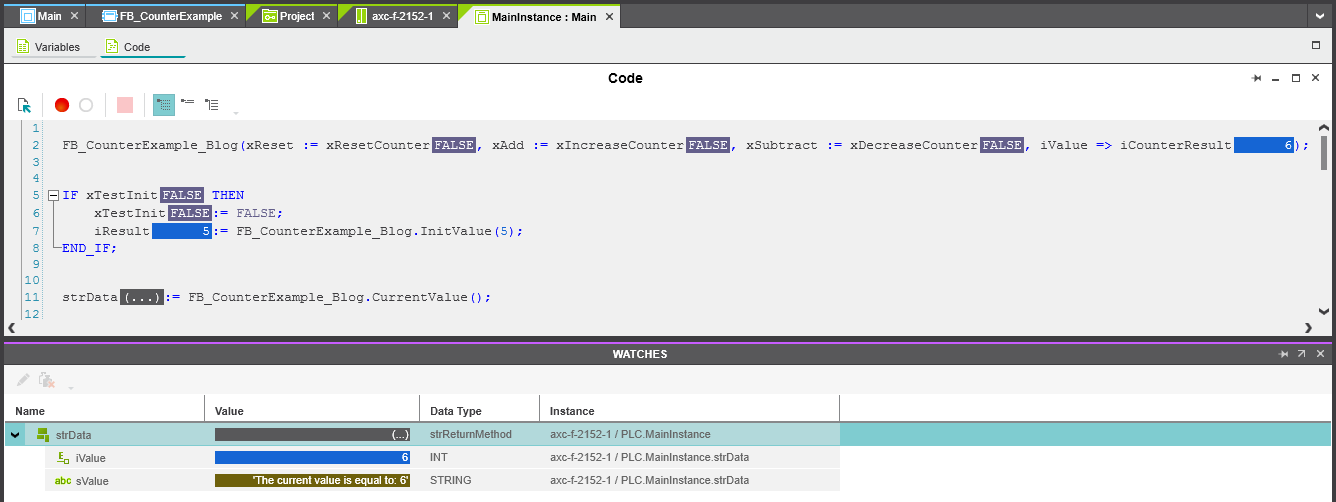## Using methods in IEC 61131-3 programming language

PLCnext Engineer supports object-oriented programming for function blocks by using methods. By adding a method to a user-defined function block POU, the function block is defined as an object-oriented function block.

To give you a good overview about methods, we will program a small function block with a counter functionality.

Add a function block with name ‘FB_CounterExample’. We need 3 input variables of type BOOL.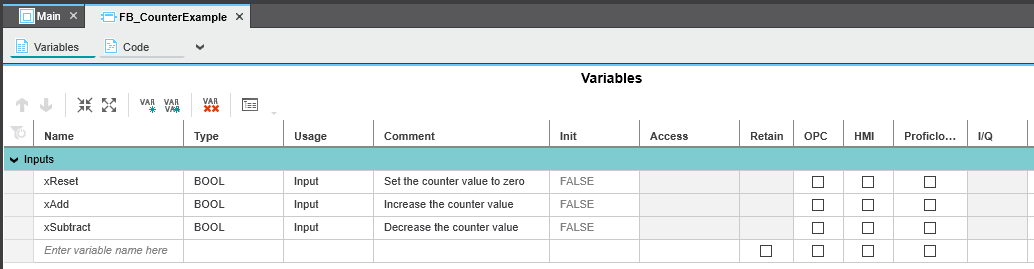To see the result of our small program, we will use an output of type INT with name iValue.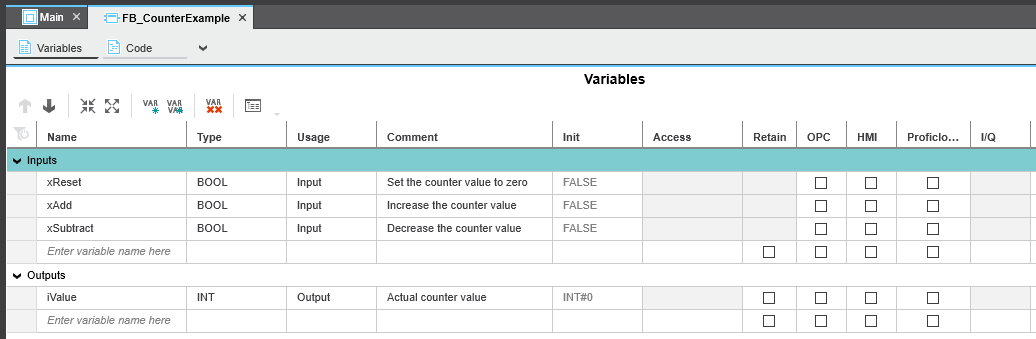Now, we are going to add the first method to increase the counter value. Right click on the function block in the components window and choose ‘Add Method’.

Double click on the method name and choose programming language ST. Now you will get a signature window. Similar to function POUs, methods do have a result type, a variable declaration part and one or more code worksheets.

For each method an access specifier must be specified. This access specifier defines from where the method may be called.

'Public': the method can be called from anywhere where it can be used (internal and external calls).

'Private': the method can only be called from inside the POU where the method is defined (internal calls only).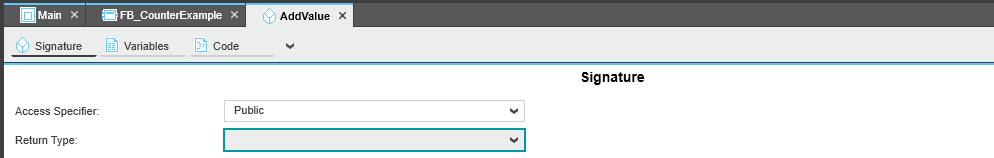For this example we select INT and Private. Now we implement the following code.

```// Increase the counter value

Now we add 2 other methods, called SubtractValue and ResetValue. Both have a return type INT and specifier Private. We need the following functionality.

```// Decrease the counter value
IF iValue > 0 THEN
SubtractValue:= iValue - 1;
ELSE
SubtractValue:= 0;
END_IF;

// Reset the counter value
ResetValue:= 0;```

Now, we are going to add some triggers in order to use the different methods. The following code should be added in the code sheet from the function block.

```R_Add(CLK := xAdd);
R_Subtract(CLK := xSubtract);
R_Reset(CLK := xReset);

END_IF;

IF R_Subtract.Q THEN
iValue:= THIS.SubtractValue();
END_IF;

IF R_Reset.Q THEN
iValue:= THIS.ResetValue();
END_IF;
```

The result should look like this. Please pay attention to the keyword ‘THIS’.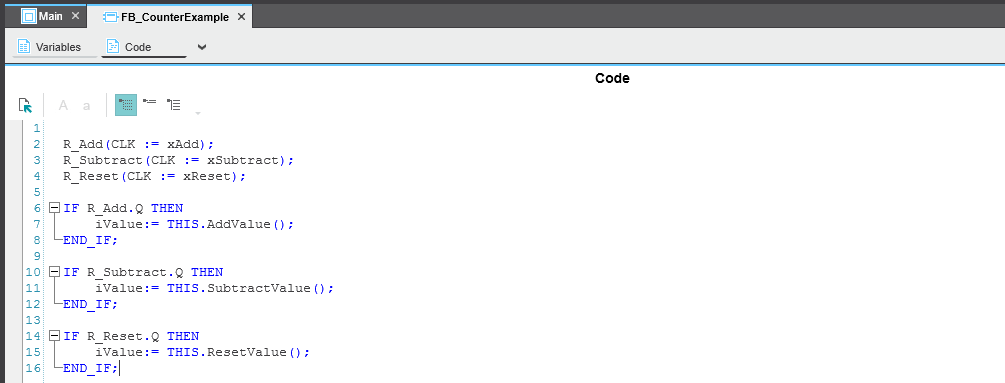Next point is to use our function block in a program ‘Main’ and have a look towards the result.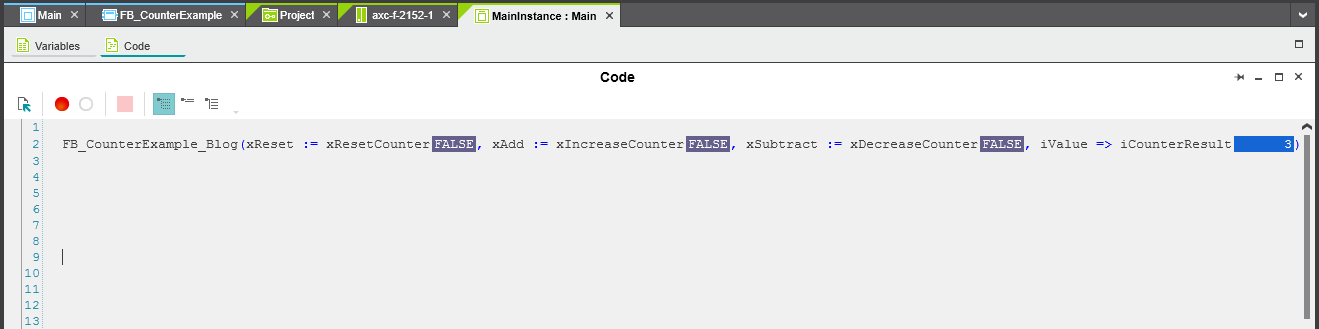Ok, so far, so good. Let’s have a look to some other possibilities. Add an extra method with name ‘InitValue’, return type INT and specifier public. Furthermore, we will give this method an input of type INT.

```// Set the value equal to the init value
InitValue:= iInitValue;
iValue:= iInitValue;
```

```IF xTestInit THEN
xTestInit:= FALSE;
iResult:= FB_CounterExample_Blog.InitValue(5);
END_IF;
```

To finish, we have one additional option left. We are going to create an own return type.

Add the following data type to your program in the component window.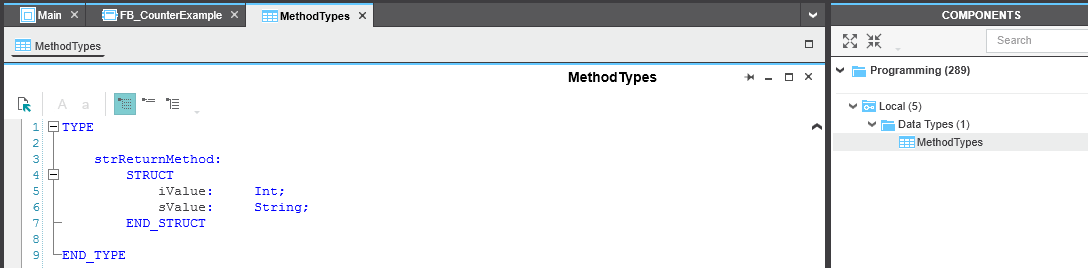We need this datatype in our last method called ‘CurrentValue’. ‘sTemp’ and ‘sValue’ are both local variables of type STRING.

```sTemp:= 'The current value is equal to: ';
sValue:= TO_STRING(iValue,'{0:d}');
sValue:= CONCAT(sTemp,sValue);
CurrentValue.iValue:= iValue;
CurrentValue.sValue:= sValue;
```

The final result: